# Semantics and complexity of GraphQL

Semantics and complexity of GraphQL Hartig & Pérez, WWW’18

(If you don’t have ACM Digital Library access, the paper can be accessed either by following the link above directly from The Morning Paper blog site, or from the WWW 2018 proceedings page).

GraphQL has been gathering good momentum since Facebook open sourced it in 2015, so I was very interested to see this paper from Hartig and Pérez exploring its properties.

One of the main advantages (of GraphQL) is its ability to define precisely the data you want, replacing multiple REST requests with a single call…

One of the most interesting questions here is what if you make a public-facing GraphQL-based API (as e.g. GitHub have done), and then the data that people ask for happens to be very expensive to compute in space and time?

Here’s a simple GraphQL query to GitHub asking for the login names of the owners of the first two repositories where ‘danbri’ is an owner.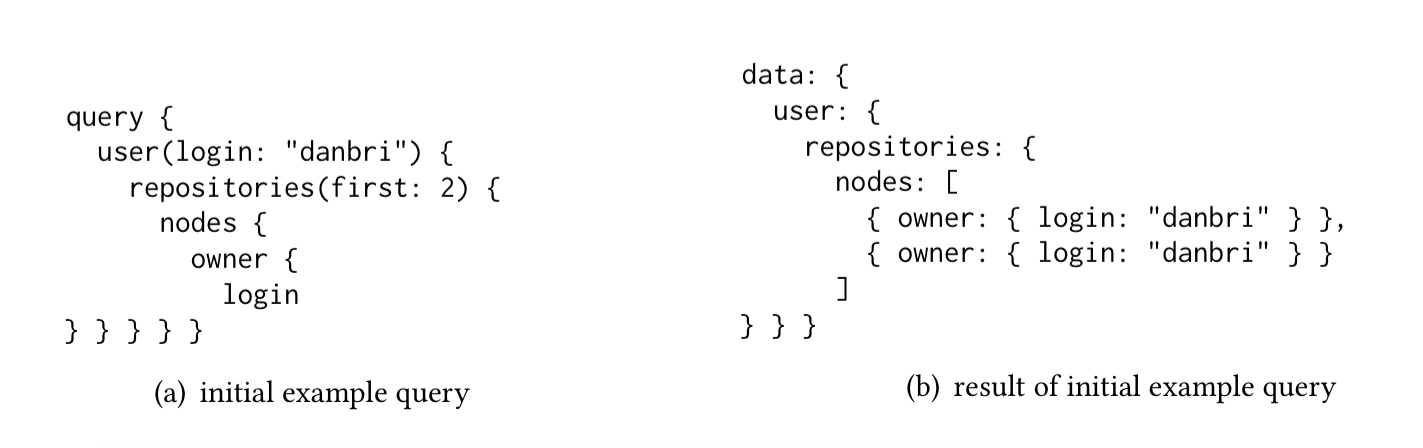From here there are two directions we can go in to expand the set of results returned : we can increase the breadth by asking for more repositories to be considered (i.e., changing first:2 to some first:n), and we can increase the depth by adding another layer of recursion (i.e., to build a level-n query we ask for the owners of the repos owned by the people returned from a level n-1 query).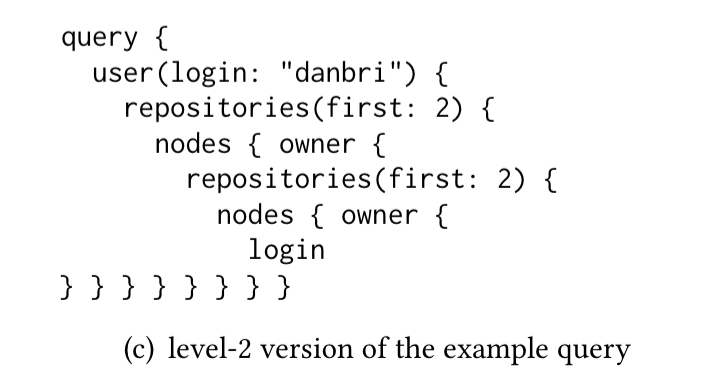Here’s what happens to the result size and execution time as you vary the level depth when considering the first 2 and the first 5 repositories: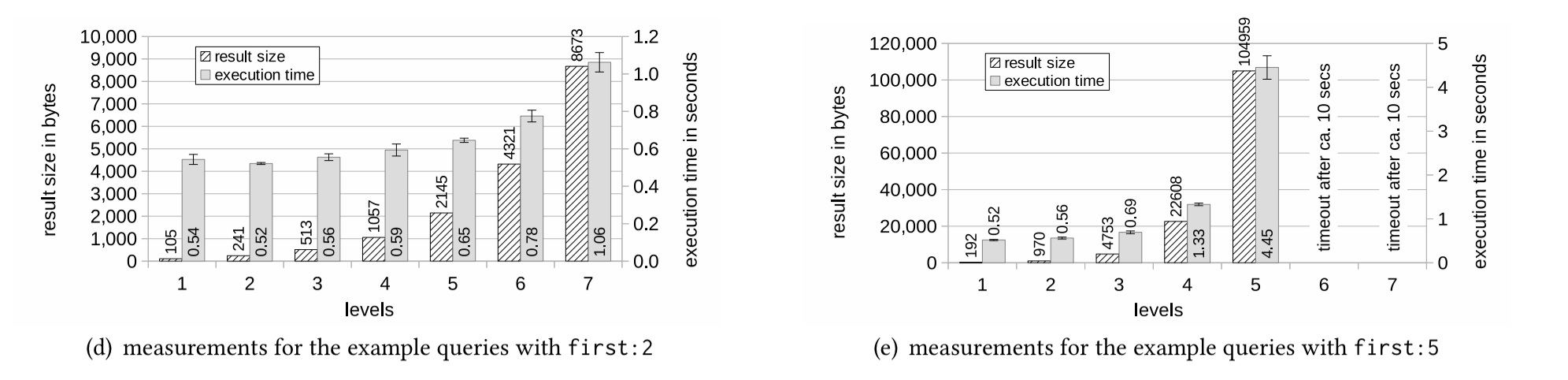You can see an exponential increase in result sizes as we increase level depth. When we ask for the first:5 repositories, the API times out at levels 6 and 7.

The response messages with those timeout errors arrive from the server a bit more than 10 seconds after issuing the requests. Hence, GitHub’s GraphQL processor clearly tries to execute these queries before their execution time exceeds a threshold. Developers have already embarked on trying to cope with this and similar issues, defining ad hoc notions of “complexity” or “cost” of GraphQL queries.

(One such mechanism is a limit on on the depth of nesting in queries, which GitHub also uses, though it wasn’t sufficient to prevent the timeout in this case). I’m sure you can spot the potential problem with allowing end user requests that can consume exponential space and time on your backend systems.

The authors study the computational complexity of GraphQL looking at three central questions:

1. The evaluation problem: what can we say about the complexity of GraphQL query evaluation?
2. The enumeration problem: how efficiently can we enumerate the results of a query in practice?
3. The response size problem: how large can responses get, and what can we do to avoid obtaining overly large response objects?

In order to do this, they need to find some solid ground to use for reasoning. So the starting point is a formalisation of the semantics of GraphQL.

### GraphQL semantics and normal form

I’m going to give only a short overview of this part of the paper, so that we can concentrate on the results that follow. GraphQL schemas are constructed over three sets: Fields (F), Arguments (A), and Types (T). Types are further subdivided into the disjoint sets object types ($O_T$), interface types ($I_T$), union types ($U_T$) and scalars.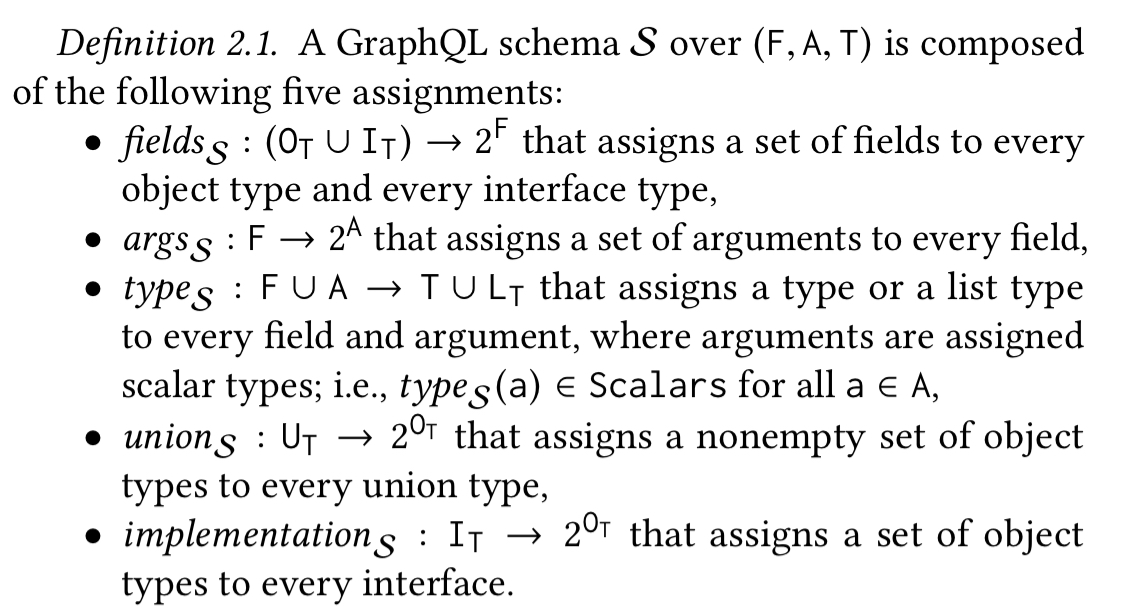(The formalisation ignores input types, non-null types, and mutation types).

GraphQL graphs are directed edge-labelled multigraphs. Each node is associated with an object type and a dictionary of properties. Property keys are field names from the set F (and a possibly empty set of argument pairs). Property values are either scalars or a sequence of scalars.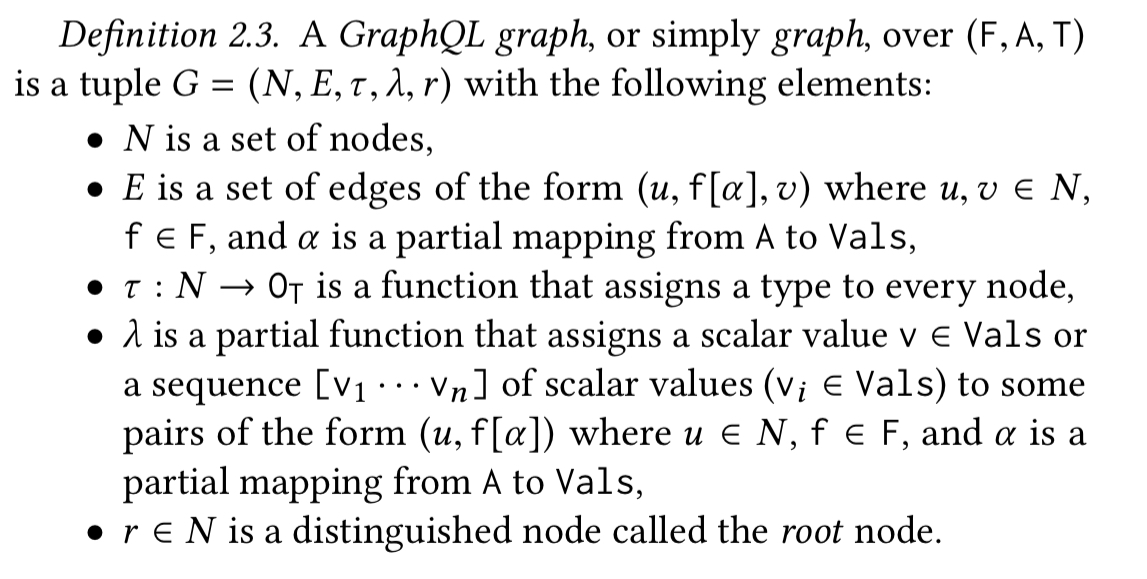The GraphQL query language lets us create expressions that can be evaluated over a graph. Queries may include sub-expressions (to be evaluated over target nodes of a parent expression), and inline fragments (on ...) aka type conditions. The paper uses$\phi$ to represent a query expression, and$|\phi|$ to denote the size of a query. The size of a query is simply the number of field selections and types that occur in it. The query below has size 11.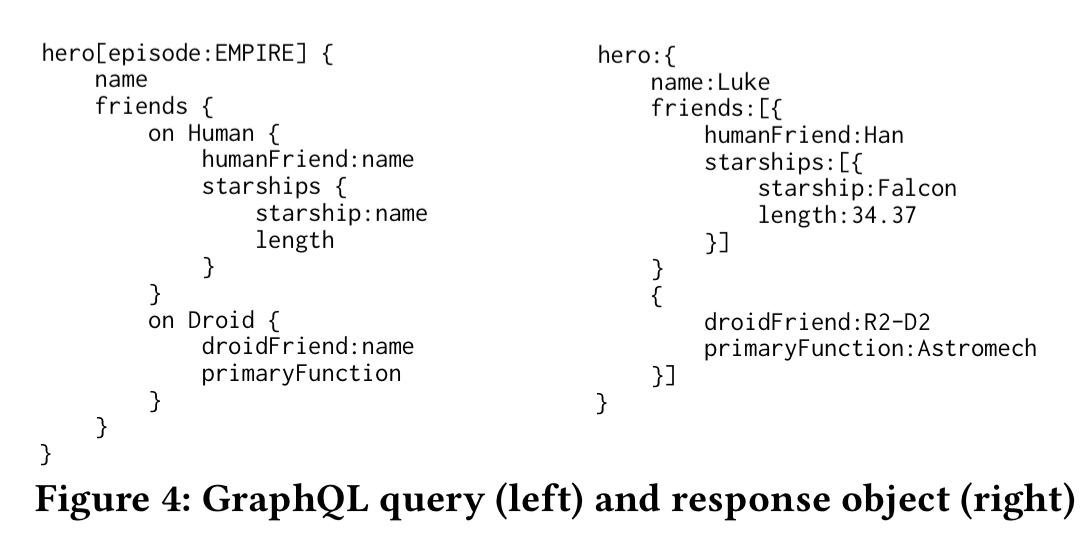It turns out to be very useful to define a ground-typed normal form for query expression, which ensures among other things that all referenced types exist in the schema. A non-redundant query has no duplication of sub-expressions. For every query conforming to some schema S we can write an equivalent non-redundant query in grounded normal form (using e.g., query-rewriting rules).

### The Evaluation problem

Let G be a GraphQL graph, u be a node, and$f[\alpha]$ be an edge label. We assume that one can access the list of$f[\alpha]$-neighbors of u in time O(1), and one can access the$f[\alpha]$-property of a node in time O(1). Although this is a standard assumption for graph databases in a RAM computational model, we stress that a GraphQL graph is usually implemented as a view over another data source and, thus, the time required to access neighbors and data may depend on the underlying data storage.

The GraphQL-Eval decision problem is this: given a graph G, a query$\phi$, and a value v, does v occur in the query results? This problem can be decided in nondeterministic logarithmic space. The sketch of the proof relies on casting the evaluation process as a series of reachability tests, each operating over tree structures which afford logspace navigation.

### The Enumeration problem

Although it is theoretically interesting to pinpoint the exact complexity of the GraphQL evaluation problem, the previous result does not give a specific hint on how the whole evaluation of a query can be actually computed in practice. We next prove that for non-redundant queries in ground-typed normal form, the complete evaluation can be done in time linear with respect to the size of the output. Actually, in proving this result we show something even stronger: the complete evaluation of a GraphQL query can be constructed symbol-by-symbol with only constant-time delay between each symbol.

The secret lies in the fact that with a non-redundant ground-typed normal form query (and recall we can rewrite any well-formed query into such a form) we don’t ever need to do field collection. (Field collection ensures that repeated fields in a query or response are not considered more than once). This means we can use a straightforward recursive ‘enumerate’ procedure.

### The Response Size problem

What all this means practically is that the size of the response is everything. If we can find ways to limit the size of the response then we can bound time and space consumption. First the bad news…

In this section we prove that even for a very simple cases [the response] object might be prohibitively large.

For a query$\phi$ over a graph G, the size of the output result has an exponential upper bound of$O(|G|^{|\phi|})$. That’s going to get big very fast indeed! This can happen both with cyclic and acyclic graphs.

A natural question at this point is how can we avoid obtaining a response object of exponential size. We prove next that we have at least two options: bound the number of different walks in the graph, or bound the nesting depth of queries. A walk in a graph is similar to a path but it is allowed to repeat edges. Notice that this implies that a graph with a cycle has an unbounded number of walks. The nesting depth of a query can be defined intuitively as the maximum number of nested curly braces in the query expression. For instance, the query in Figure 4 (above) has nesting depth 4.

These are somewhat crude tools though: both can cause us to reject queries that would have accommodate an efficient evaluation, and to still accept queries for which a complete evaluation is too resource intensive. For example, GitHub restrict nesting to 25 levels, but we saw earlier a query that will timeout with just 6.

We can do better!

Fortunately, we can give a more elegant solution by showing that the exact size of the complete evaluation of a GraphQL query can be computed efficiently without the need of evaluating the whole query.

Algorithms 1 and 2 below show how this can be done in polynomial time.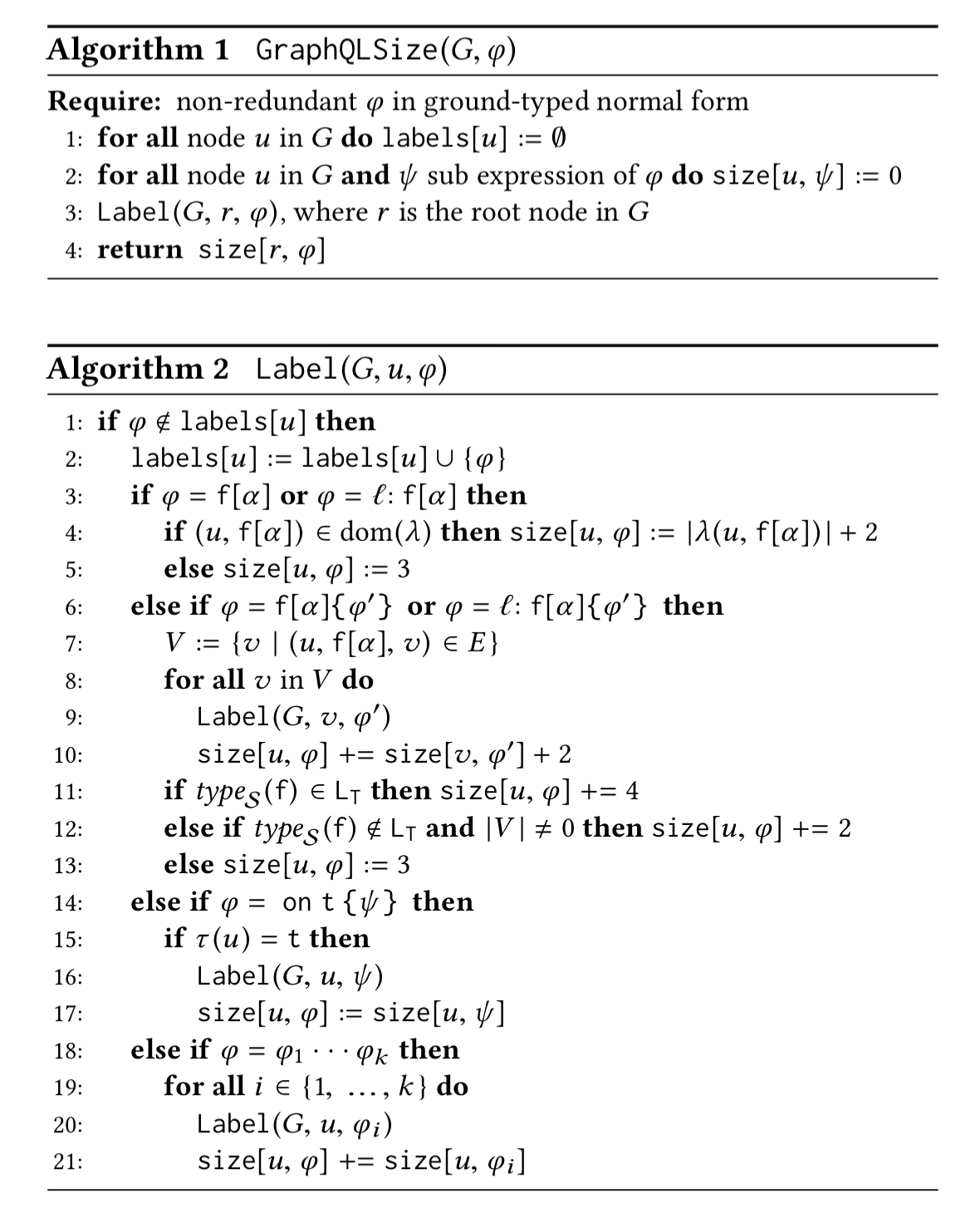Putting it all together, you can build a robust GraphQL API by rewriting incoming queries into non-redundant ground-typed normal form, computing the expected result size in polynomial time, and then proceeding to execute the query only if the expected size is below an acceptable threshold.